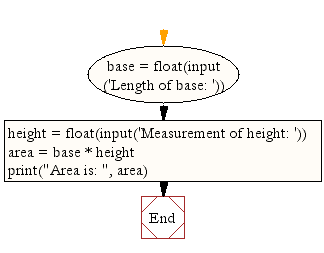﻿ Python Math: Calculate the area of a parallelogram - w3resource# Python Math: Calculate the area of a parallelogram

## Python Math: Exercise-4 with Solution

Write a Python program to calculate the area of a parallelogram.
Note: A parallelogram is a quadrilateral with opposite sides parallel (and therefore opposite angles equal). A quadrilateral with equal sides is called a rhombus, and a parallelogram whose angles are all right angles is called a rectangle.

Sample Solution:-

Python Code:

``````base = float(input('Length of base: '))
height = float(input('Measurement of height: '))
area = base * height
print("Area is: ", area)
```
```

Sample Output:

```Length of base: 5
Measurement of height: 6
Area is:  30.0
```

Pictorial Presentation:Flowchart:## Visualize Python code execution:

The following tool visualize what the computer is doing step-by-step as it executes the said program:

Python Code Editor:

Have another way to solve this solution? Contribute your code (and comments) through Disqus.

What is the difficulty level of this exercise?

Test your Programming skills with w3resource's quiz.

﻿

## Python: Tips of the Day

Performs right-to-left function composition:

Example:

```from functools import reduce

def tips_compose(*fns):
return reduce(lambda f, g: lambda *args: f(g(*args)), fns)
add6 = lambda x: x + 6
multiply = lambda x, y: x * y
```36